Tuesday 3rd August 2021# Classroom Key Stage 2 / KS2 - Maths Activities & Resources

## Welcome to the Maths section of the Key Stage 2 classroom. Within KS2 Maths you will find online @school assessment tests that will help children to enhance their learning and revise the subject of Maths at Key Stage 2 level.

•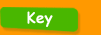•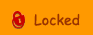••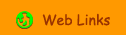•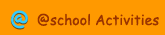•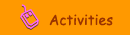•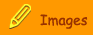###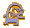Number & The Number System

Decimal Line - Level 6 - Interactive test to recognise decimals.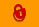Decimals - Level 6 - Interactive test to order decimal numbers.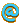Decimals - Level 6 - 2 - Interactive test about decimal numbers.Dienes Blocks - Interactive activity where you need to use Dienes blocks to represent numbers - younger KS2 - revision.Finding Fractions - Interactive activity where you have to find the fraction for a group of objects.Missing Digits - Interactive test about inserting a three digit number.Missing Numbers - 2 - Interactive test to find missing numbers in a number grid.Missing Sequence - Interactive test to find the missing numbers in a sequence.Number - Interactive test about making a number using four digits.Number - 1 - Interactive test to solve problems using 3 digits.Number Order - Interactive test to order large numbers starting with the smallest first.Number Sequence - Find the missing number in this sequence with this interactive test.Number Sequence - Level 6 - Interactive test about the sequence of numbers for level 6 children.Number Think - Level 6 - Interactive test to find the original number - for level 6 children.Positive and Negative Numbers - Interactive test about calculating negative and positive numbers.Sequences - 1 - Find the missing number in this online sequence test.Sequences - 2 - Interactive test to find the missing numbers in this sequence including negative numbers.Sequencing - Interactive test to find the numbers in these sequences.Sequencing - Interactive activity about sequencing numbers.